# High School Math : Right Triangles

## Example Questions

1 2 3 4 5 6 7 8 9 11 Next →

### Example Question #3 : Triangles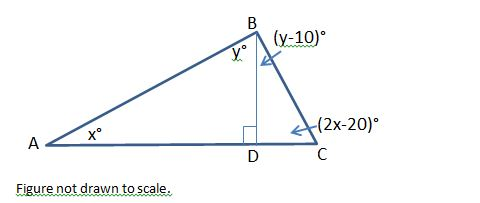In the figure above, what is the positive difference, in degrees, between the measures of angle ACB and angle CBD?

20

10

40

30

50

10

Explanation:

In the figure above, angle ADB is a right angle. Because side AC is a straight line, angle CDB must also be a right angle.

Let’s examine triangle ADB. The sum of the measures of the three angles must be 180 degrees, and we know that angle ADB must be 90 degrees, since it is a right angle. We can now set up the following equation.

x + y + 90 = 180

Subtract 90 from both sides.

x + y = 90

Next, we will look at triangle CDB. We know that angle CDB is also 90 degrees, so we will write the following equation:

y – 10 + 2x – 20 + 90 = 180

y + 2x + 60 = 180

Subtract 60 from both sides.

y + 2x = 120

We have a system of equations consisting of x + y = 90 and y + 2x = 120. We can solve this system by solving one equation in terms of x and then substituting this value into the second equation. Let’s solve for y in the equation x + y = 90.

x + y = 90

Subtract x from both sides.

y = 90 – x

Next, we can substitute 90 – x into the equation y + 2x = 120.

(90 – x) + 2x = 120

90 + x = 120

x = 120 – 90 = 30

x = 30

Since y = 90 – x, y = 90 – 30 = 60.

The question ultimately asks us to find the positive difference between the measures of ACB and CBD. The measure of ACB = 2x – 20 = 2(30) – 20 = 40 degrees. The measure of CBD = y – 10 = 60 – 10 = 50 degrees. The positive difference between 50 degrees and 40 degrees is 10.

### Example Question #1 : How To Find An Angle In A Right Triangle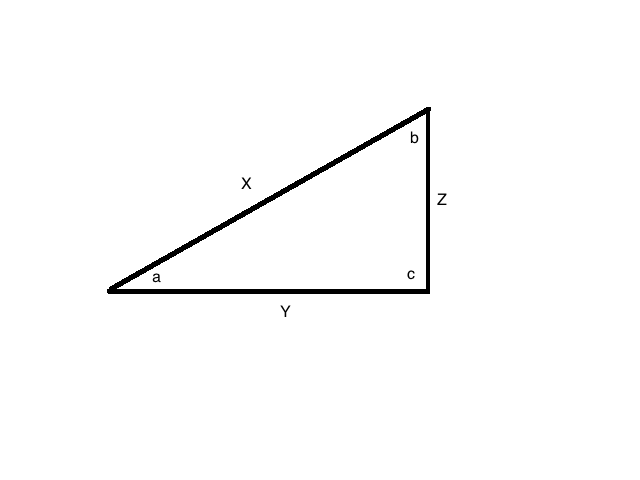If angle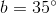and angle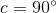, what is the value for angle?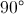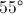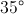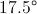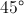Explanation:

For this problem, remember that the sum of the degrees in a triangle is.

That means that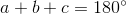.

Plug in our given values to solve: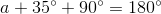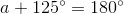Subtract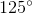from both sides: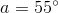### Example Question #4 : Triangles

Which of the following sets of line-segment lengths can form a triangle?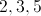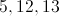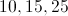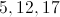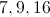Explanation:

In any given triangle, the sum of any two sides is greater than the third.  The incorrect answers have the sum of two sides equal to the third.

### Example Question #1 : How To Find An Angle In A Right Triangle

In right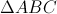,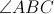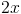and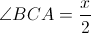.

What is the value of?

48

32

30

24

36

36

Explanation:

There are 180 degrees in every triangle. Since this triangle is a right triangle, one of the angles measures 90 degrees.

Therefore,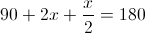.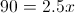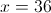1 2 3 4 5 6 7 8 9 11 Next →